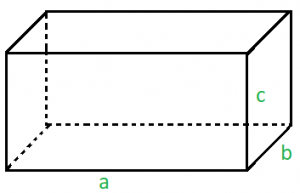# Sum of lengths of all 12 edges of any rectangular parallelepiped

Given the area of three faces of the rectangular parallelepiped which has a common vertex. Our task is to find the sum of lengths of all 12 edges of this parallelepiped.

In geometry, a parallelepiped is a three-dimensional figure formed by six parallelograms. By analogy, it relates to a parallelogram just as a cube relates to a square or as a cuboid to a rectangle. A picture of a rectangular parallelepiped is shown below.Examples:

Input: 1 1 1
Output: 12

Input: 20 10 50
Output: 68


## Recommended: Please try your approach on {IDE} first, before moving on to the solution.

Approach: The area given are s1, s2 and s3 . Let a, b and c be the lengths of the sides that have one common vertex. Where,,. It’s easy to find the length in terms of faces areas:,,. The answer will be the summation of all the 4 sides, there are four sides that have lengths equal to a, b and c.

In the first example the given area s1 = 1, s2 = 1 and s3 = 1. So with the above approach, the value of a, b, c will come out to be 1. So the sum of the length of all 12 edges will be 4 * 3 = 12.

Below is the implementation of the above approach:

## C++

 // C++ program to illustrate  // the above problem  #include  using namespace std;     // function to find the sum of  // all the edges of parallelepiped  double findEdges(double s1, double s2, double s3)  {      // to calculate the length of one edge      double a = sqrt(s1 * s2 / s3);      double b = sqrt(s3 * s1 / s2);      double c = sqrt(s3 * s2 / s1);         // sum of all the edges of one side      double sum = a + b + c;         // net sum will be equal to the      // summation of edges of all the sides      return 4 * sum;  }     // Driver code  int main()  {      // initialize the area of three      // faces which has a common vertex      double s1, s2, s3;      s1 = 65, s2 = 156, s3 = 60;         cout << findEdges(s1, s2, s3);         return 0;  }

## Java

 // Java program to illustrate  // the above problem     import java.io.*;     class GFG {       // function to find the sum of  // all the edges of parallelepiped  static double findEdges(double s1, double s2, double s3)  {      // to calculate the length of one edge      double a = Math.sqrt(s1 * s2 / s3);      double b = Math.sqrt(s3 * s1 / s2);      double c = Math.sqrt(s3 * s2 / s1);         // sum of all the edges of one side      double sum = a + b + c;         // net sum will be equal to the      // summation of edges of all the sides      return 4 * sum;  }            // Driver code         public static void main (String[] args) {              // initialize the area of three      // faces which has a common vertex      double s1, s2, s3;      s1 = 65; s2 = 156; s3 = 60;         System.out.print(findEdges(s1, s2, s3));      }  }        // this code is contributed by anuj_67..

## Python3

 import math     # Python3 program to illustrate  # the above problem     # function to find the sum of  # all the edges of parallelepiped  def findEdges(s1, s2, s3):         # to calculate the length of one edge      a = math.sqrt(s1 * s2 / s3)      b = math.sqrt(s3 * s1 / s2)      c = math.sqrt(s3 * s2 / s1)         # sum of all the edges of one side      sum = a + b + c         # net sum will be equal to the      # summation of edges of all the sides      return 4 * sum       # Driver code  if __name__=='__main__':         # initialize the area of three  # faces which has a common vertex      s1 = 65     s2 = 156     s3 = 60        print(int(findEdges(s1, s2, s3)))             # This code is contributed by   # Shivi_Aggarwal

## C#

 // C# program to illustrate  // the above problem  using System;     public class GFG{         // function to find the sum of  // all the edges of parallelepiped  static double findEdges(double s1, double s2, double s3)  {      // to calculate the length of one edge      double a = Math.Sqrt(s1 * s2 / s3);      double b = Math.Sqrt(s3 * s1 / s2);      double c = Math.Sqrt(s3 * s2 / s1);         // sum of all the edges of one side      double sum = a + b + c;         // net sum will be equal to the      // summation of edges of all the sides      return 4 * sum;  }     // Driver code         static public void Main (){      // initialize the area of three      // faces which has a common vertex      double s1, s2, s3;      s1 = 65; s2 = 156; s3 = 60;         Console.WriteLine(findEdges(s1, s2, s3));      }  }        // This code is contributed by anuj_67..

## PHP

 

Output:

120


Don’t stop now and take your learning to the next level. Learn all the important concepts of Data Structures and Algorithms with the help of the most trusted course: DSA Self Paced. Become industry ready at a student-friendly price.

My Personal Notes arrow_drop_upCheck out this Author's contributed articles.

If you like GeeksforGeeks and would like to contribute, you can also write an article using contribute.geeksforgeeks.org or mail your article to contribute@geeksforgeeks.org. See your article appearing on the GeeksforGeeks main page and help other Geeks.

Please Improve this article if you find anything incorrect by clicking on the "Improve Article" button below.

Article Tags :
Practice Tags :

Be the First to upvote.

Please write to us at contribute@geeksforgeeks.org to report any issue with the above content.